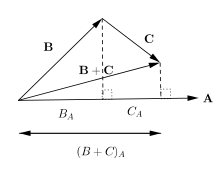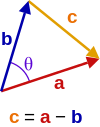# Dot product

"Scalar product" redirects here. For the abstract scalar product, see Inner product space. For the product of a vector and a scalar, see Scalar multiplication.

In mathematics, the dot product or scalar product (sometimes inner product in the context of Euclidean space, or rarely projection product for emphasizing the geometric significance), is an algebraic operation that takes two equal-length sequences of numbers (usually coordinate vectors) and returns a single number. This operation can be defined either algebraically or geometrically. Algebraically, it is the sum of the products of the corresponding entries of the two sequences of numbers. Geometrically, it is the product of the Euclidean magnitudes of the two vectors and the cosine of the angle between them. The name "dot product" is derived from the centered dot " · " that is often used to designate this operation; the alternative name "scalar product" emphasizes that the result is a scalar (rather than a vector).

In three-dimensional space, the dot product contrasts with the cross product of two vectors, which produces a pseudovector as the result. The dot product is directly related to the cosine of the angle between two vectors in Euclidean space of any number of dimensions.

## Definition

The dot product may be defined algebraically or geometrically. The geometric definition is based on the notions of angle and distance (magnitude of vectors). The equivalence of these two definitions relies on having a Cartesian coordinate system for Euclidean space.

In modern presentations of Euclidean geometry, the points of space are defined in terms of their Cartesian coordinates, and Euclidean space itself is commonly identified with the real coordinate space Rn. In such a presentation, the notions of length and angles are not primitive. They are defined by means of the dot product: the length of a vector is defined as the square root of the dot product of the vector by itself, and the cosine of the (non oriented) angle of two vectors of length one is defined as their dot product. So the equivalence of the two definitions of the dot product is a part of the equivalence of the classical and the modern formulations of Euclidean geometry.

### Algebraic definition

The dot product of two vectors a = [a1, a2, ..., an] and b = [b1, b2, ..., bn] is defined as:where Σ denotes summation notation and n is the dimension of the vector space. For instance, in three-dimensional space, the dot product of vectors [1, 3, −5] and [4, −2, −1] is:### Geometric definition

In Euclidean space, a Euclidean vector is a geometrical object that possesses both a magnitude and a direction. A vector can be pictured as an arrow. Its magnitude is its length, and its direction is the direction that the arrow points. The magnitude of a vector a is denoted by. The dot product of two Euclidean vectors a and b is defined bywhere θ is the angle between a and b.

In particular, if a and b are orthogonal, then the angle between them is 90° andAt the other extreme, if they are codirectional, then the angle between them is 0° andThis implies that the dot product of a vector a by itself iswhich givesthe formula for the Euclidean length of the vector.

### Scalar projection and first propertiesScalar projection

The scalar projection (or scalar component) of a Euclidean vector a in the direction of a Euclidean vector b is given bywhere θ is the angle between a and b.

In terms of the geometric definition of the dot product, this can be rewrittenwhereis the unit vector in the direction of b.Distributive law for the dot product

The dot product is thus characterized geometrically byThe dot product, defined in this manner, is homogeneous under scaling in each variable, meaning that for any scalar α,It also satisfies a distributive law, meaning thatThese properties may be summarized by saying that the dot product is a bilinear form. Moreover, this bilinear form is positive definite, which means thatis never negative and is zero if and only if### Equivalence of the definitions

If e1, ..., en are the standard basis vectors in Rn, then we may writeThe vectors ei are an orthonormal basis, which means that they have unit length and are at right angles to each other. Hence since these vectors have unit lengthand since they form right angles with each other, if ij,Thus in general we can say that:Where δ ij is the Kronecker delta.

Also, by the geometric definition, for any vector ei and a vector a, we notewhere ai is the component of vector a in the direction of ei.

Now applying the distributivity of the geometric version of the dot product giveswhich is precisely the algebraic definition of the dot product. So the (geometric) dot product equals the (algebraic) dot product.

## Properties

The dot product fulfills the following properties if a, b, and c are real vectors and r is a scalar.

1. Commutative:which follows from the definition (θ is the angle between a and b):3. Bilinear:4. Scalar multiplication:5. Not associative because the dot product between a scalar (a ⋅ b) and a vector (c) is not defined, which means that the expressions involved in the associative property, (a ⋅ b) ⋅ c or a ⋅ (b ⋅ c) are both ill-defined. Note however that the previously mentioned scalar multiplication property is sometimes called the "associative law for scalar and dot product" or one can say that "the dot product is associative with respect to scalar multiplication" because c (ab) = (c a) ⋅ b = a ⋅ (c b).
6. Orthogonal:
Two non-zero vectors a and b are orthogonal if and only if ab = 0.
7. No cancellation:
Unlike multiplication of ordinary numbers, where if ab = ac, then b always equals c unless a is zero, the dot product does not obey the cancellation law:
If ab = ac and a0, then we can write: a ⋅ (bc) = 0 by the distributive law; the result above says this just means that a is perpendicular to (bc), which still allows (bc) ≠ 0, and therefore bc.
8. Product Rule: If a and b are functions, then the derivative (denoted by a prime ′) of ab is a′ ⋅ b + ab.

### Application to the law of cosinesTriangle with vector edges a and b, separated by angle θ.
Main article: Law of cosines

Given two vectors a and b separated by angle θ (see image right), they form a triangle with a third side c = ab. The dot product of this with itself is:which is the law of cosines.

## Triple product expansion

Main article: Triple product

This is an identity (also known as Lagrange's formula) involving the dot- and cross-products. It is written as:which may be remembered as "BAC minus CAB", keeping in mind which vectors are dotted together. This formula finds application in simplifying vector calculations in physics.

## Physics

In physics, vector magnitude is a scalar in the physical sense, i.e. a physical quantity independent of the coordinate system, expressed as the product of a numerical value and a physical unit, not just a number. The dot product is also a scalar in this sense, given by the formula, independent of the coordinate system. Examples include:

## Generalizations

### Complex vectors

For vectors with complex entries, using the given definition of the dot product would lead to quite different properties. For instance the dot product of a vector with itself would be an arbitrary complex number, and could be zero without the vector being the zero vector (such vectors are called isotropic); this in turn would have consequences for notions like length and angle. Properties such as the positive-definite norm can be salvaged at the cost of giving up the symmetric and bilinear properties of the scalar product, through the alternative definitionwhere bi is the complex conjugate of bi. Then the scalar product of any vector with itself is a non-negative real number, and it is nonzero except for the zero vector. However this scalar product is thus sesquilinear rather than bilinear: it is conjugate linear and not linear in b, and the scalar product is not symmetric, sinceThe angle between two complex vectors is then given byThis type of scalar product is nevertheless useful, and leads to the notions of Hermitian form and of general inner product spaces.

### Inner product

Main article: Inner product space

The inner product generalizes the dot product to abstract vector spaces over a field of scalars, being either the field of real numbersor the field of complex numbers. It is usually denoted by.

The inner product of two vectors over the field of complex numbers is, in general, a complex number, and is sesquilinear instead of bilinear. An inner product space is a normed vector space, and the inner product of a vector with itself is real and positive-definite.

### Functions

The dot product is defined for vectors that have a finite number of entries. Thus these vectors can be regarded as discrete functions: a length-n vector u is, then, a function with domain {k ∈ ℕ ∣ 1 ≤ kn}, and ui is a notation for the image of i by the function/vector u.

This notion can be generalized to continuous functions: just as the inner product on vectors uses a sum over corresponding components, the inner product on functions is defined as an integral over some interval axb (also denoted [a, b]):Generalized further to complex functions ψ(x) and χ(x), by analogy with the complex inner product above, gives### Weight function

Inner products can have a weight function, i.e. a function which weight each term of the inner product with a value.

Matrices have the Frobenius inner product, which is analogous to the vector inner product. It is defined as the sum of the products of the corresponding components of two matrices A and B having the same size:(For real matrices)

### Tensors

The inner product between a tensor of order n and a tensor of order m is a tensor of order n + m − 2, see tensor contraction for details.

## Computation

### Algorithms

The straightforward algorithm for calculating a floating-point dot product of vectors can suffer from catastrophic cancellation. To avoid this, approaches such as the Kahan summation algorithm are used.

### Libraries

A dot product function is included in BLAS level 1.

## References

1. The term scalar product is often also used more generally to mean a symmetric bilinear form, for example for a pseudo-Euclidean space.
2. S. Lipschutz; M. Lipson (2009). Linear Algebra (Schaum’s Outlines) (4th ed.). McGraw Hill. ISBN 978-0-07-154352-1.
3. M.R. Spiegel; S. Lipschutz; D. Spellman (2009). Vector Analysis (Schaum’s Outlines) (2nd ed.). McGraw Hill. ISBN 978-0-07-161545-7.
4. A I Borisenko; I E Taparov (1968). Vector and tensor analysis with applications. Translated by Richard Silverman. Dover. p. 14.
5. Arfken, G. B.; Weber, H. J. (2000). Mathematical Methods for Physicists (5th ed.). Boston, MA: Academic Press. pp. 14–15. ISBN 978-0-12-059825-0..
6. Weisstein, Eric W. "Dot Product." From MathWorld--A Wolfram Web Resource. http://mathworld.wolfram.com/DotProduct.html
7. T. Banchoff; J. Wermer (1983). Linear Algebra Through Geometry. Springer Science & Business Media. p. 12. ISBN 978-1-4684-0161-5.
8. A. Bedford; Wallace L. Fowler (2008). Engineering Mechanics: Statics (5th ed.). Prentice Hall. p. 60. ISBN 978-0-13-612915-8.
9. K.F. Riley; M.P. Hobson; S.J. Bence (2010). Mathematical methods for physics and engineering (3rd ed.). Cambridge University Press. ISBN 978-0-521-86153-3.
10. M. Mansfield; C. O’Sullivan (2011). Understanding Physics (4th ed.). John Wiley & Sons. ISBN 978-0-47-0746370.